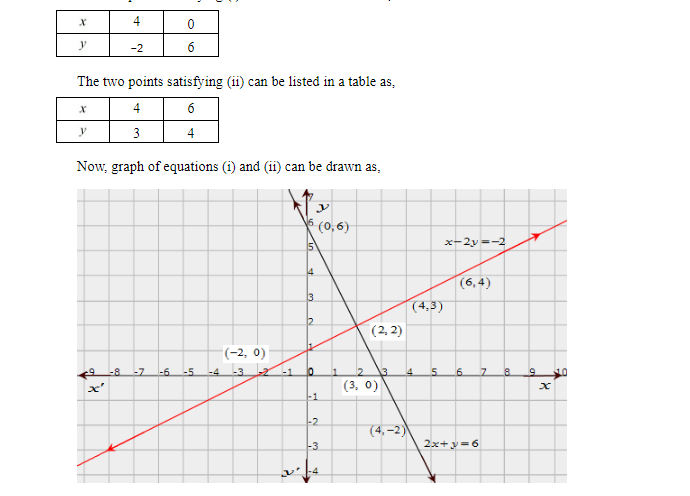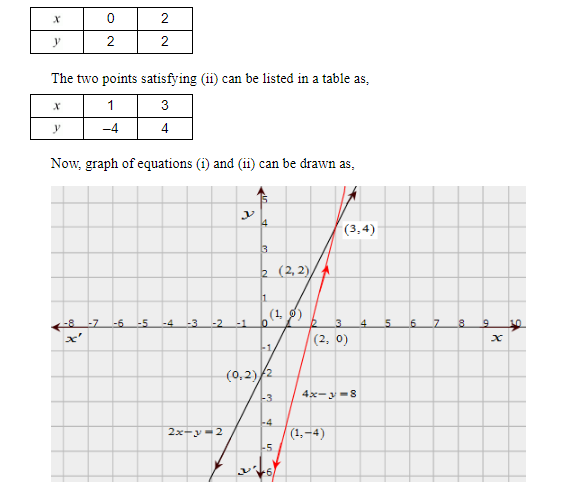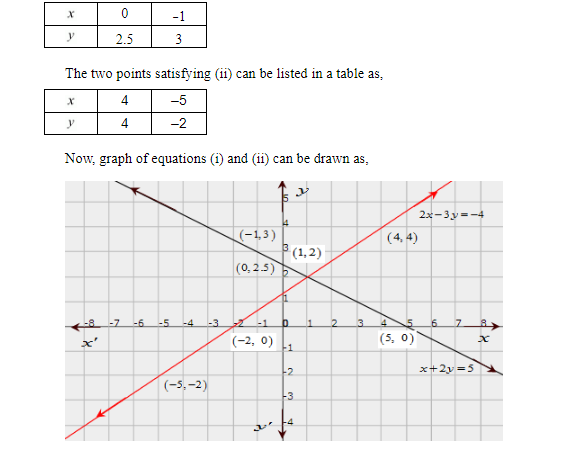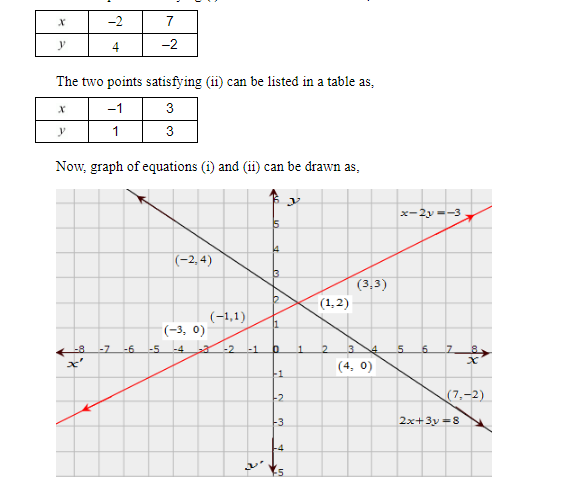# Solve graphically each of the following systems of linear equations. Also, find the coordinates of the points where the lines meet the axis of x in each system.

Question:

Solve graphically each of the following systems of linear equations. Also, find the coordinates of the points where the lines meet the axis of x in each system.

(i) $2 x+y=6$

$x-2 y=-2$

(ii) $2 x-y=2$

$4 x-y=8$

(iii) $x+2 y=5$

$2 x-3 y=-4$

(iv) $2 x+3 y=8$

$x-2 y=-3$

Solution:

(i)

The given equations are

$2 x+y=6$...(i)

$x-2 y=-2$....(ii)

The two points satisfying (i) can be listed in a table as,It is seen that the solution of the given system of equations is given by x = 2, y = 2.

Also, it is observed that the coordinates of the points where the lines (i) and (ii) meet the $x$-axis are $(3,0)$ and $(-2,0)$ respectively.

(ii) The given equations are

$2 x-y=2$....(i)

$4 x-y=8$....(ii)

The two points satisfying (i) can be listed in a table as,It is seen that the solution of the given system of equations is given by x = 3, y = 4.

Also, it is observed that the coordinates of the points where the lines (i) and (ii) meet the x-axis are $(1,0)$ and $(2,0)$ respectively.

(iii) The given equations are

$x+2 y=5$ ...(i)

$2 x-3 y=-4$....(ii)

The two points satisfying (i) can be listed in a table as,It is seen that the solution of the given system of equations is given by x = 1, y = 2.

Also, it is observed that the coordinates of the points where the lines (i) and (ii) meet the $x$-axis are $(5,0)$ and $(-2,0)$ respectively.

Solution is missing

(iv) The given equations are

$2 x+3 y=8$....(i)

$x-2 y=-3$....(ii)

The two points satisfying (i) can be listed in a table as,It is seen that the solution of the given system of equations is given by x = 1, y = 2.

Also, it is observed that the coordinates of the points where the lines (i) and (ii) meet the x-axis are $(4,0)$ and $(-3,0)$ respectively.